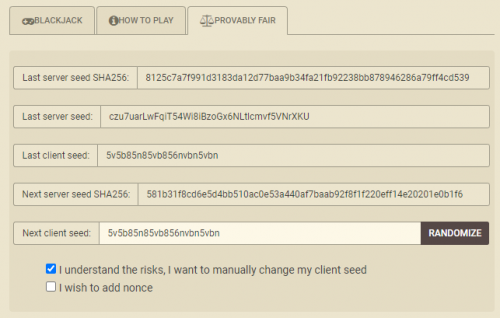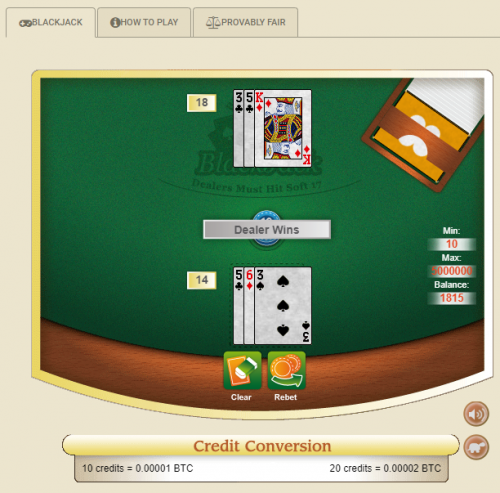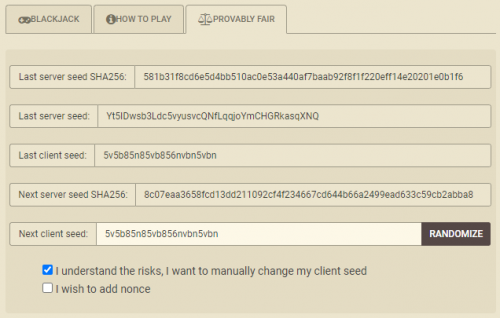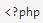Last Updated: July 2, 2020

# Blackjack (Encrypted Version)

## Introduction

This page is about the encrypted version of blackjack, often played at casinos that are based in cryptocurrency. I assume the reader is already familiar with the basic rules of conventional blackjack.

### Provably Fair Casinos

Name Wizards Seal VA Friendly  Play
YES YES

## Analysis

In the case of the game at Crypto.Games, the bizarre set of rules are as follows:

• 4 decks
• Shuffle after each hand
• Dealer does not peek for blackjack
• Blackjack pays 6:5
• Dealer hits on Soft 17
• Double on any two cards
• Hit split aces
• Early surrender
• A ten and ace after splitting counts as blackjack
• Double after split allowed
• Player may split once only (I assume)

Not considering early surrender and 10-A after splitting counting as a blackjack, my blackjack house edge calculator gives a house edge of 1.91% with basic strategy. However, my list of blackjack rule variations, says the value of the two rules the calculator doesn't support is 0.74%. This makes the overall house edge 1.17%, which is quite high for an online blackjack game.

## Fair Gaming

Following is the process Crypto.Games uses to randomly choose cards from a four-deck shoe for their blackjack game.

1. Reveal to player the SHA-256 Hash of the Server Seed before the bet is made.
2. After the bet is made, combine the Server Seed and Client Seed, in that order.
3. Take the SHA-512 Hash of the combined seed from step 1.
4. Take two characters from the Hash found in step 2, staring at the left.
5. Convert the two characters from step 3 from hexadecimal to decimal. If you don't understand what I'm talking about, I go over base-16 math in my page on Dice (Encrypted Version).
6. If the result of step 4 is 207 or less, then map that card number to a specific card in the 208-card shoe.
7. If that card number has not been found yet in the hand, then map it to a particular playing card. Here is how to do that:
• To get the rank, divide the card number by 13 and take the remainder. Map the remainder to a rank, as follows: 0 to ace, 1 to 2, 2 to 3, 3 to 4, 4 to 5, 5 to 6, 6 to 7, 7 to 8, 8 to 9, 9 to 10, 10 to jack, 11 to queen, 12 to king.
• To get the suit, divide the card number by 13 and drop the remainder. Then, divide that quotient by 4 and take the remainder. Then take that remainder and map it to a suit, as follows: 0 to spades, 1 to hearts, 2 to diamonds, 3 to clubs.
8. Otherwise, if the result of step 5 was greater than 207, or that card had been found already, then ignore those two digits in the Hash.
9. Advance two positions in the Hash and then go back to step 3, until you reach the end of the Hash. That should give you many more cards than you'll need to process a hand of blackjack.

## Example

Following is how the process works for an example hand.The image above, from the Fair Gaming panel, was taken before making a bet. The import things to do to ensure a fair game are to enter and record a new Client Seed (a bunch of random characters will do) and the Hash of the next Server Seed, which the game titles "Next server seed SHA256." Here they are:

• Client seed = 5v5b85n85vb856nvbn5vbn

• Next server seed SHA256 = `581b31f8cd6e5d4bb510ac0e53a440af7baab92f8f1f220eff14e20201e0b1f6`

Personally, I copy and paste these items into Notepad, if go through this process the hard way.In the image above I start with a bet of 0.00001 BTC (Bitcoin), which is equivalent to about 10¢. They make the player use this bet to by ten chips and then bet the whole ten. Why they muddy the water with this step, I don't know.

My initial hand was a total of 11 against a three, so I doubled, and got a 3. The dealer had a 5 in the hole, for a total of 8, then drew a king, for a total of 18. Thus, I lost 14 to 18.Next, I go back to the Fair Gaming panel to ensure the game was fair, as evidenced by the order of cards being predestined before I made the bet. Following the steps above:

1. From the Fair Gaming panel, we see the Server Seed was `Yt5IDwsb3Ldc5vyusvcQNfLqqjoYmCHGRkasqXNQ`.
2. We take the SHA-256 Hash of that to get 581b31f8cd6e5d4bb510ac0e53a440af7baab92f8f1f220eff14e20201e0b1f6.
3. We compare the Hash from step 2 to the "Next server seed SHA256" recorded before the bet and verify they match. If they don't, either you did something wrong, or the casino is lying/cheating. In this case, they match. This verifies the order of cards was predestined.
4. To check you got the right cards, combine the Server Seed and Client Seed, in that order. That will give you Yt5IDwsb3Ldc5vyusvcQNfLqqjoYmCHGRkasqXNQ5v5b85n85vb856nvbn5vbn. If you chose to have a nonce, that is at the end of the Client Seed.
5. Take the SHA-512 Hash of the combined Hash from step 4. Why they use two different Hashing functions (SHA-256 and SHA-512), I have no idea. This will give you 2b87fbc5eac7368ef393c8ab292f71a0251702f6db028ca8a855bfaa541b78df3ae996ad22ac022588a46231ed32180d5cbde86dff5d2368cbb7658332bbb9bc.
6. Take the first two characters, which are 2b, and convert them to hexadecimal: 2b = 2*16 + 11 = 43.
7. Since 43 <= 207, convert it to a card: rank = mod(43,13) = 4, which maps to a 5. The suit = mod(int(43/13),4) = mod(3,4) = 3, which maps to clubs. This is the first card dealt to the player, the 5 of clubs.
8. Advance two positions in the Hash from step 4, which are 87. Convert that from hexadecimal to decimal: 8*16 + 7 = 135.
9. Since 135 <= 207 and hasn't been found yet, convert it to a card: rank = mod(135,13) = 5, which maps to a 6. The suit = mod(int(135/13),4) = mod(10,4) = 2, which maps to diamonds. This is the second card dealt to the player, the 6 of diamonds.
10. Advance two positions in the Hash from step 4, which are fb. Convert that from hexadecimal to decimal: 15*16 + 11 = 251.
11. Since 251 > 207, we skip those two positions.
12. Advance two positions in the Hash from step 4, which are c5. Convert that from hexadecimal to decimal: 12*16 + 5 = 197.
13. Since 197 <= 207 and hasn't been found yet, convert it to a card: rank = mod(197,13) = 2, which maps to a rank of 3. The suit = mod(int(197/13),4) = mod(15,4) = 3, which maps to clubs. This is the first (face up) card dealt to the dealer, the 3 of clubs.
14. Advance two positions in the Hash from step 4, which are ea. Convert that from hexadecimal to decimal: 14*16 + 10 = 234.
15. Since 234 > 207, we skip those two positions.
16. Advance two positions in the Hash from step 4, which are c7. Convert that from hexadecimal to decimal: 12*16 + 7 = 199.
17. Since 199 <= 207 and hasn't been found yet, convert it to a card: rank = mod(199,13) = 4, which maps to a 5. The suit = mod(int(199/13),4) = mod(15,4) = 3, which maps to clubs. This is the second (face down) card dealt to the dealer, the 5 of clubs.
18. Advance two positions in the Hash from step 4, which are 36. Convert that from hexadecimal to decimal: 3*16 + 6 = 54.
19. Since 54 <= 207 and hasn't been found yet, convert it to a card: rank = mod(54,13) = 2, which maps to a 3. The suit = mod(int(54/13),4) = mod(4,4) = 0, which maps to spades. This, the 3 of spades, is the next card in the shoe, which went to the player after the double down. The player's total is thus 5+6+3 = 14
20. Advance two positions in the Hash from step 4, which are 8e. Convert that from hexadecimal to decimal: 8*16 + 14 = 142.
21. Since 142 <= 207 and hasn't been found yet, convert it to a card: rank = mod(142,13) = 12, which maps to a king. The suit = mod(int(142/13),4) = mod(10,4) = 2, which maps to diamonds. This, the king of diamonds, is the next card in the shoe, which went to the dealer when he had to hit a hard 8, getting a total of 18.
22. The player loses 14 to 18.
23. If more cards were required, we would keep looping through this process until enough cards were found.

If that seems like a lot of steps to jump through, I agree. That is why I wrote a PHP script to do it for you! To use it, following these steps:

1. Go to the PHP Sandbox.
2. Enter the Server Seed on line 3.
3. Enter the Client Seed on line 4.
4. Enter the Hash of the next Server Seed on line 5.
5. Click "Execute Code."

The program will very the Server Seed hashes to what was provided before you bet and the cards dealt in the game.

I also have a copy of the code you may see by clicking the button below.```// blackjack fairness check for Crypto.Games
\$server_seed = "Yt5IDwsb3Ldc5vyusvcQNfLqqjoYmCHGRkasqXNQ";
\$client_seed = "5v5b85n85vb856nvbn5vbn";
\$next_hash = "581b31f8cd6e5d4bb510ac0e53a440af7baab92f8f1f220eff14e20201e0b1f6";
\$rank_array=array("A",2,3,4,5,6,7,8,9,10,"J","Q","K");
\$cards_found=0;
\$position=0;
\$combined_seed = \$server_seed.\$client_seed;
echo "Combined seed = \$combined_seed\n";
\$combined_hash = hash('sha512', \$combined_seed);
echo "Hash of combined seed = \$combined_hash\n";
do
{
\$first_two=substr(\$combined_hash,\$position,2);
\$hex_to_dec=hexdec(\$first_two);
if (\$hex_to_dec <=207)
{
\$repeat=0;
if (\$cards_found>0)
{
for (\$i=0; \$i<\$cards_found; \$i++)
{
if ( \$hex_to_dec == \$card_array[\$i])
{ \$repeat=1; }
}
}
if (\$repeat==0)
{
\$card_array[\$cards_found] = \$hex_to_dec;
\$cards_found++;
\$rank=\$hex_to_dec%13;
\$suit=intdiv(\$hex_to_dec,13)%4;
echo "Card \$cards_found = \t\$rank_array[\$rank] of \$suit_array[\$suit]\n";
}
}
\$position+=2;
if (\$position==128)
{
echo "Error -- No more space in hash.\n";
\$cards_found=10;
}
}
while (\$cards_found<20);
\$server_seed_hash=hash('sha256', \$server_seed);
if (\$server_seed_hash==\$next_hash)
{ echo "Server Seed match.\n"; }
else
{
echo "SERVER SEED MISMATCH!\n";
echo "Server seed      =\t\$server_seed\n";
echo "Server seed Hash =\t\$server_seed_hash\n";
echo "Alleged next Hash=\t\$next_hash\n";
}

// Procedure
// 1.  Step the "step" equal to 0 and the "position" to 0.
// 2.  Join server and client seeds,step, and server seed, in that order.
// 3.  Generate a SHA-512 hash of the string from step 2.
// 4.  Convert first two characters, starting at the "position" of the hash from step 3 from hexidecimal to decimal.
// 5.  If the result from step 4 is 0 to 207, then map it to a specific card in a four-deck shoe.
// 6.  If the result from step 5 has appeared yet, then that shall be the next card to be dealt in the game.
// 7.  To get a card position from step 6 to an actual card, do as follows:
//     A.  Divide the result from step 4 by 13 and take the remainder.
//     B.  Map the result from step 7A to get the rank, as follows: 0 to A, 1 to 2, 2 to 3, ... , 9 to 10, 10 to J, 11 to Q, 12 to K.
//     C.  Divide the result from step 4 by 13 and DROP the remainer.
//     D.  Divide the result from step 7C by 4 and take the remainder.
//     E.  Map the result from step 7D to get the suit, as follows: 0 to spades, 1 to hearts, 2 to diamonds, 3 to clubs.
// 8.  Keep repeating steps 4 to 7 until you reach the end of the hash, although it is unlikely this many cards will be needed in the game.
?>
```

Written by: